# RD Sharma Solutions for Class 8 Maths Chapter 16 Understanding Shapes- II (Quadrilaterals)

We have learnt the angle sum property of a triangle in the earlier class. In Chapter 16 of Class 8, we shall learn concepts based on the angle sum property of a quadrilateral and other polygons. These concepts are explained in detail and exercise-wise solutions are prepared by our expert faculty team in the best possible ways students can understand. Students are advised to refer to RD Sharma Class 8 as reference material, which helps in boosting their confidence level to achieve high marks in their exams. The students can also easily download the PDFs for free from the links provided below.

Chapter 16- Understanding Shapes-II (Quadrilaterals) contains one exercise and the RD Sharma Solutions present in this page provide solutions to the questions present in this exercise. Now, let us have a look at the concepts discussed in this chapter.

• Definitions of the terms- sides, adjacent sides, opposite sides, diagonals and angles.
• Interior and exterior of a quadrilateral.
• Interior angle sum property.
• Exterior angle property.

## Download the Pdf of RD Sharma Solutions for Class 8 Maths Chapter 16 Understanding Shapes- II (Quadrilaterals)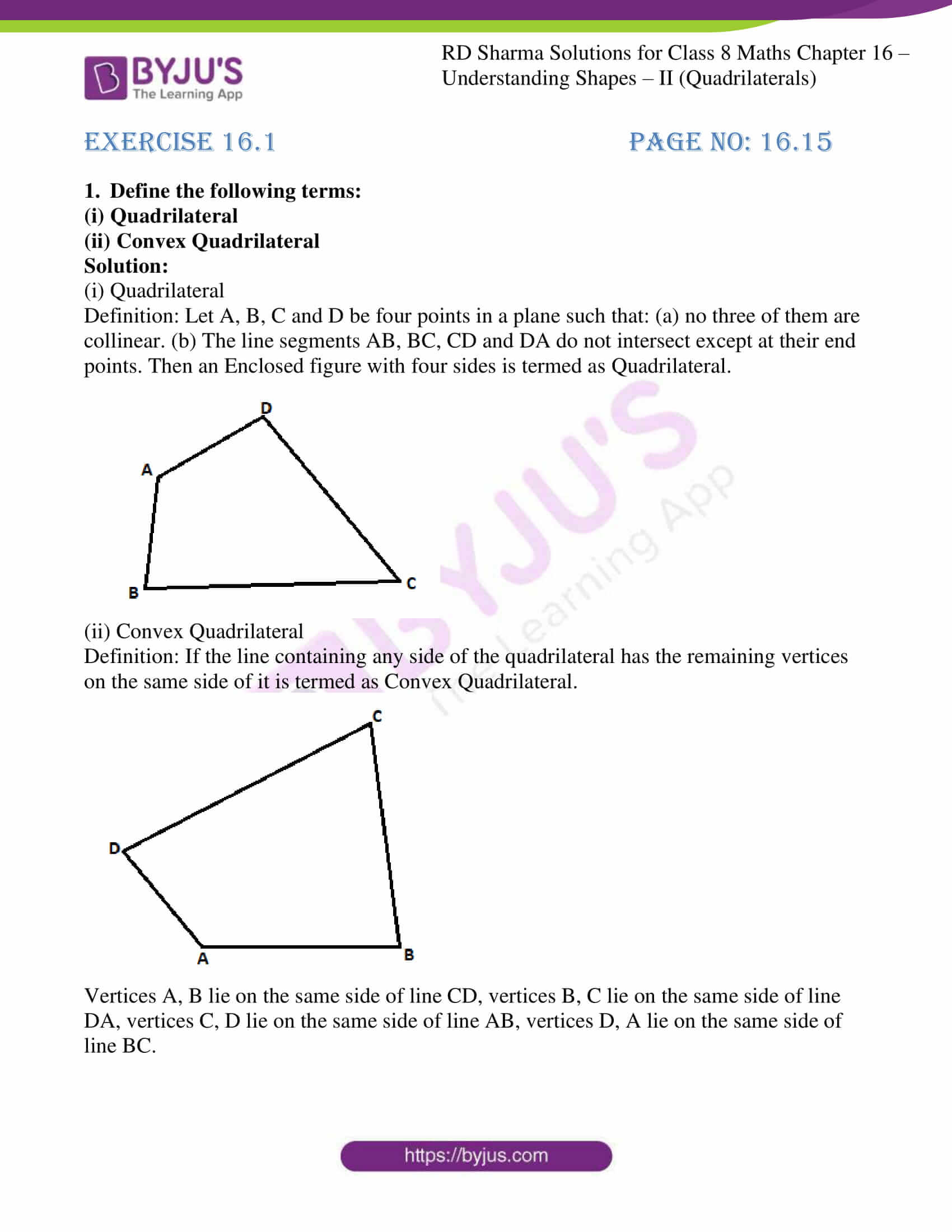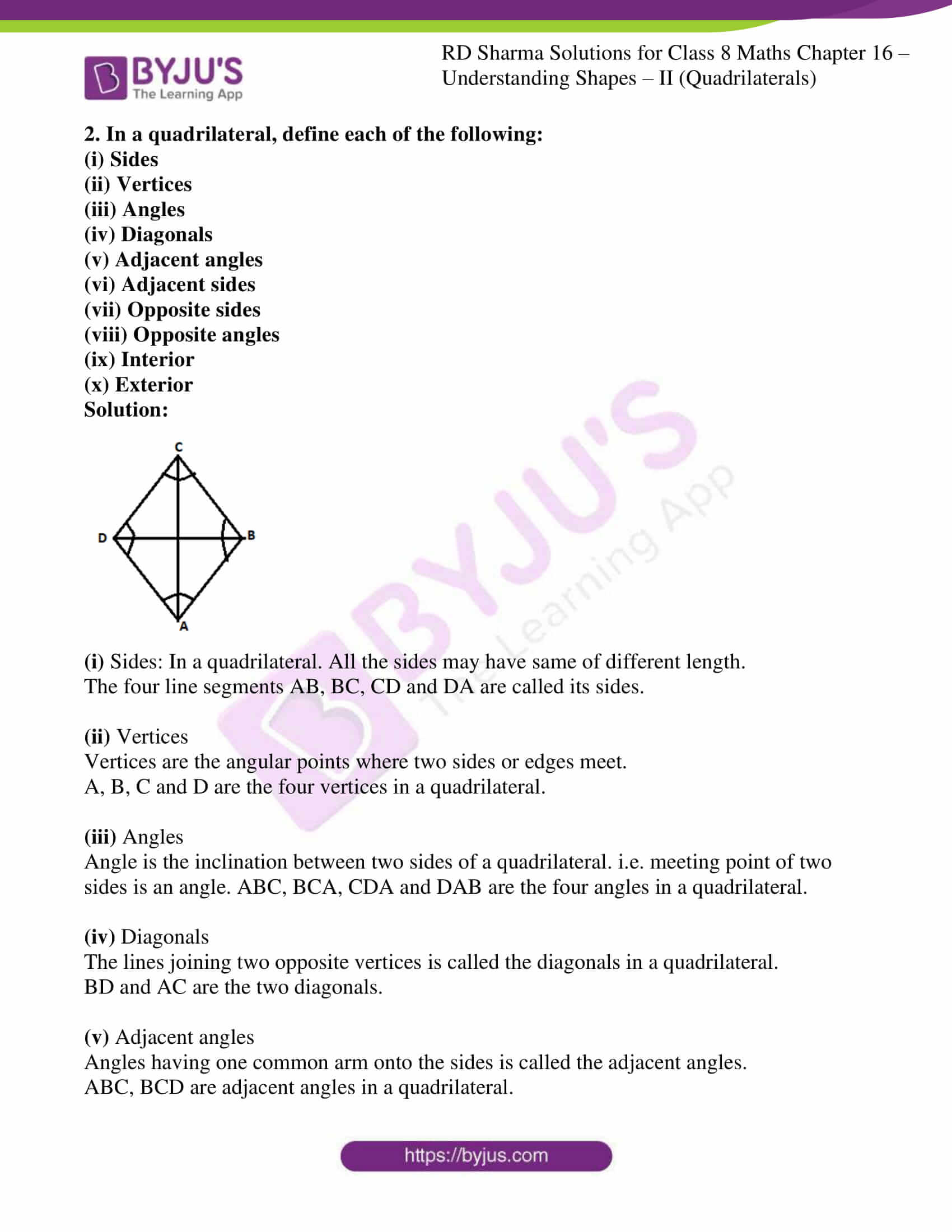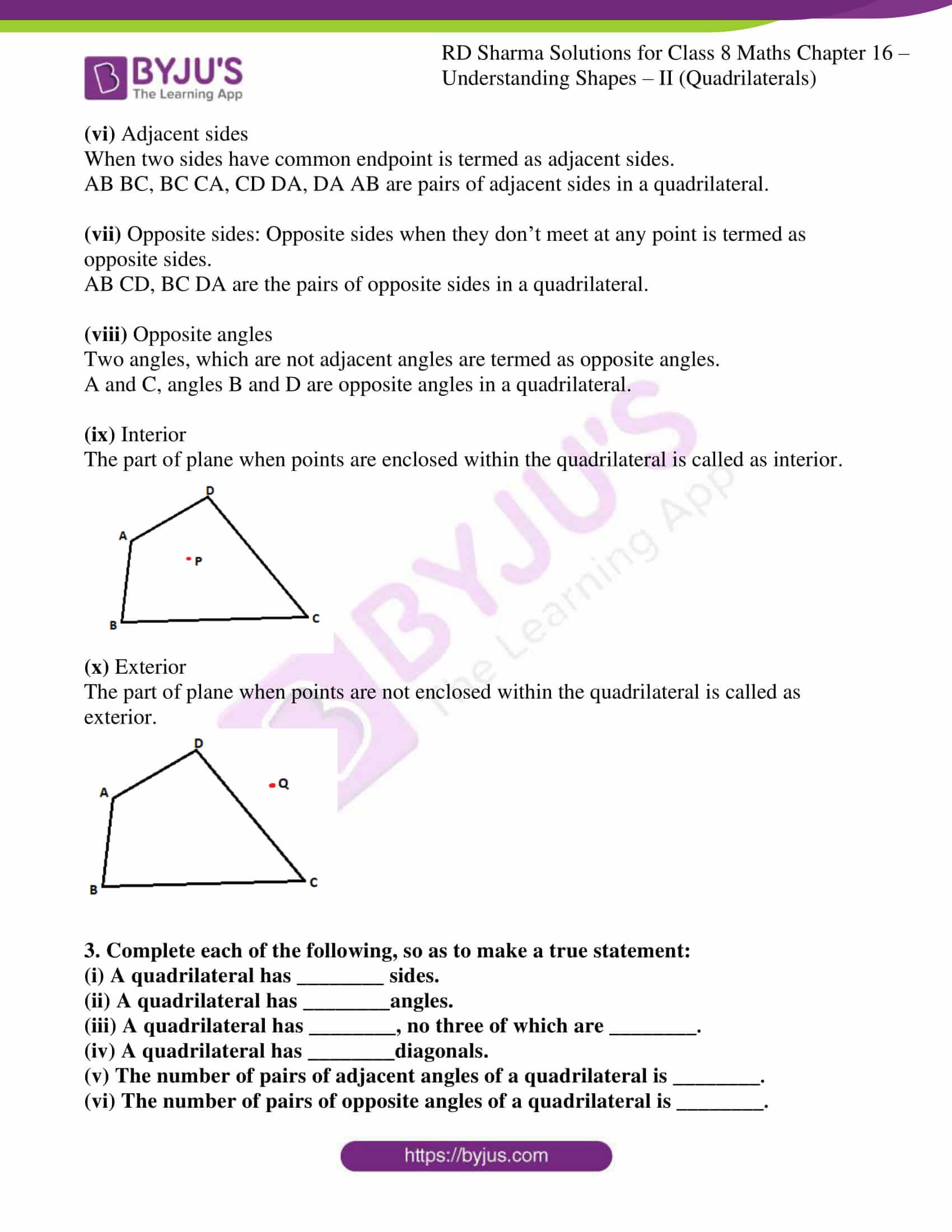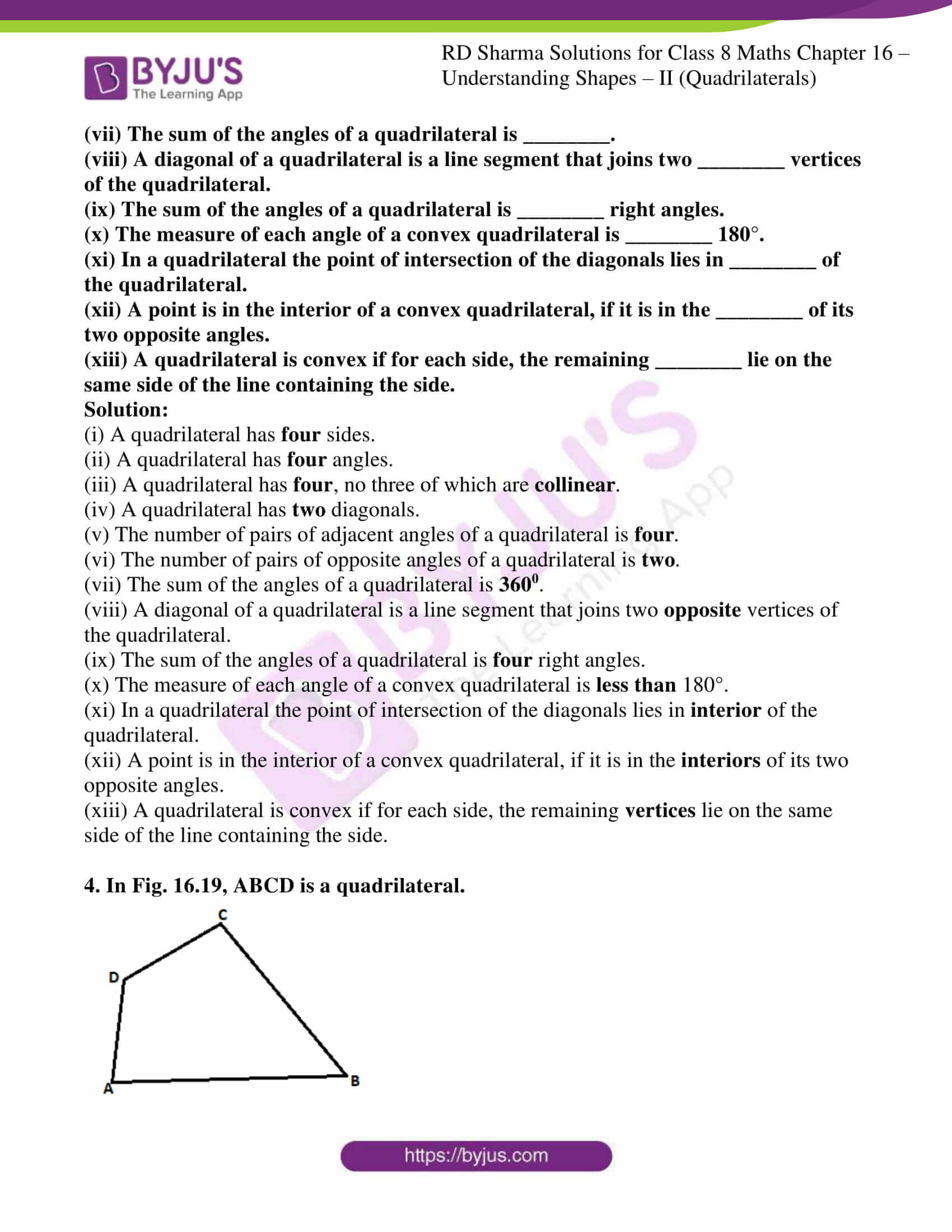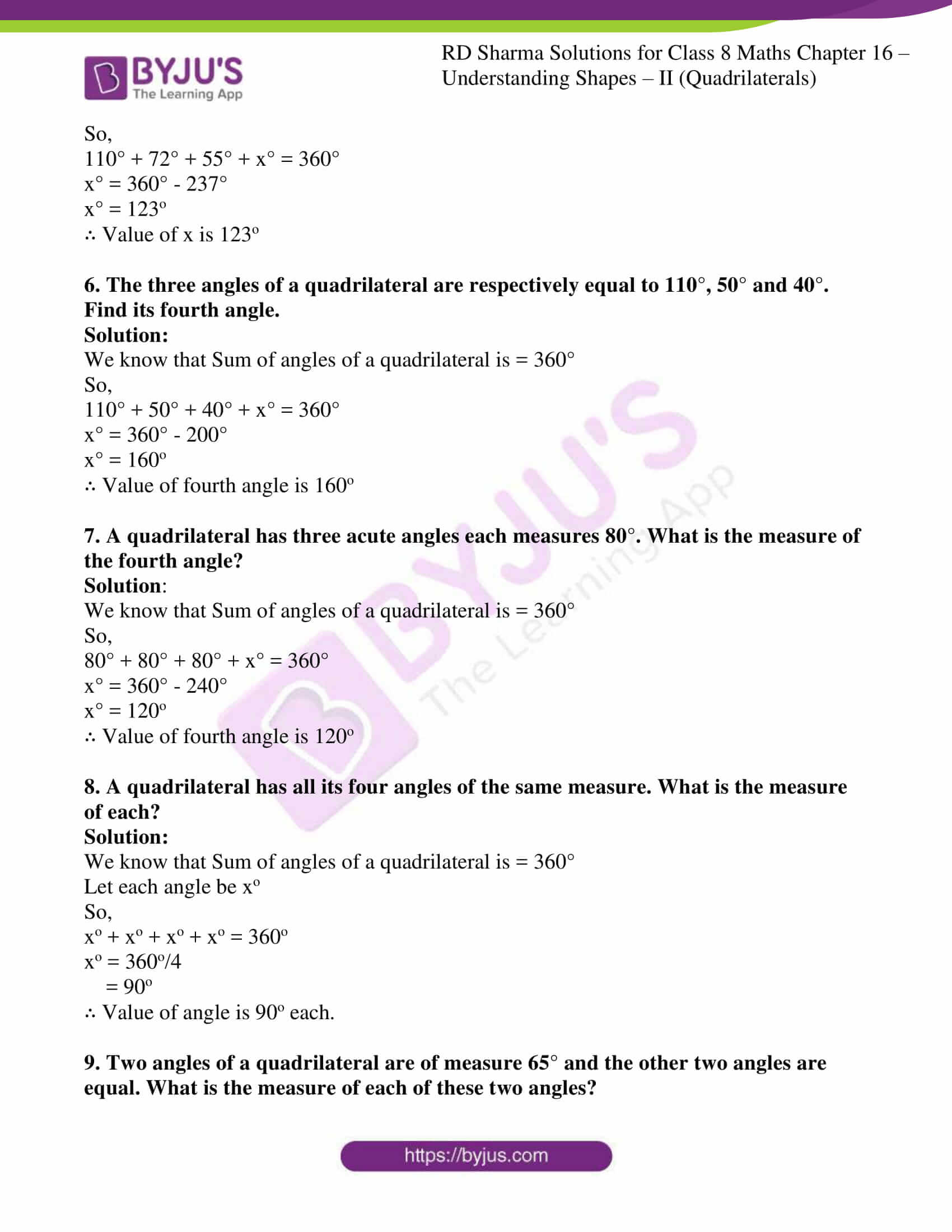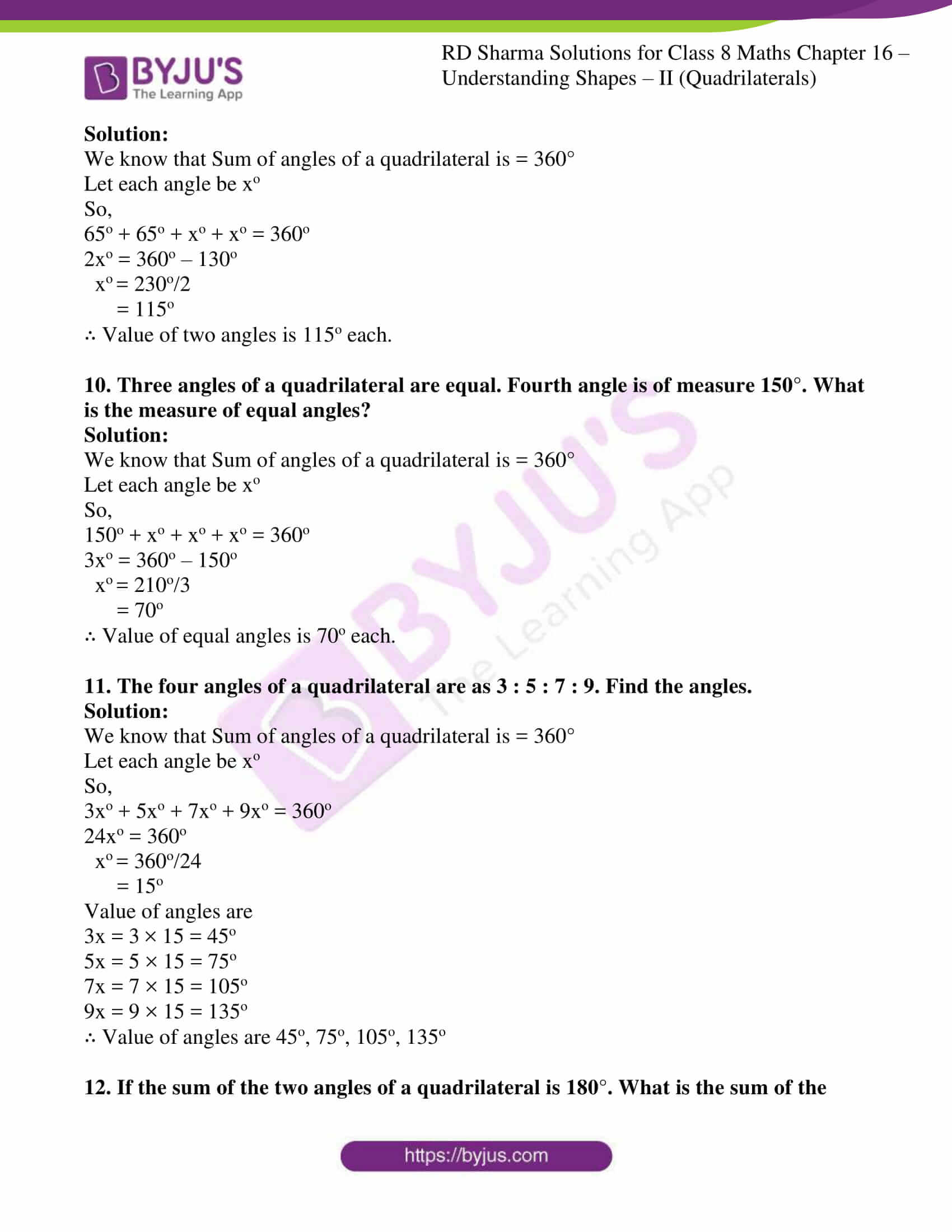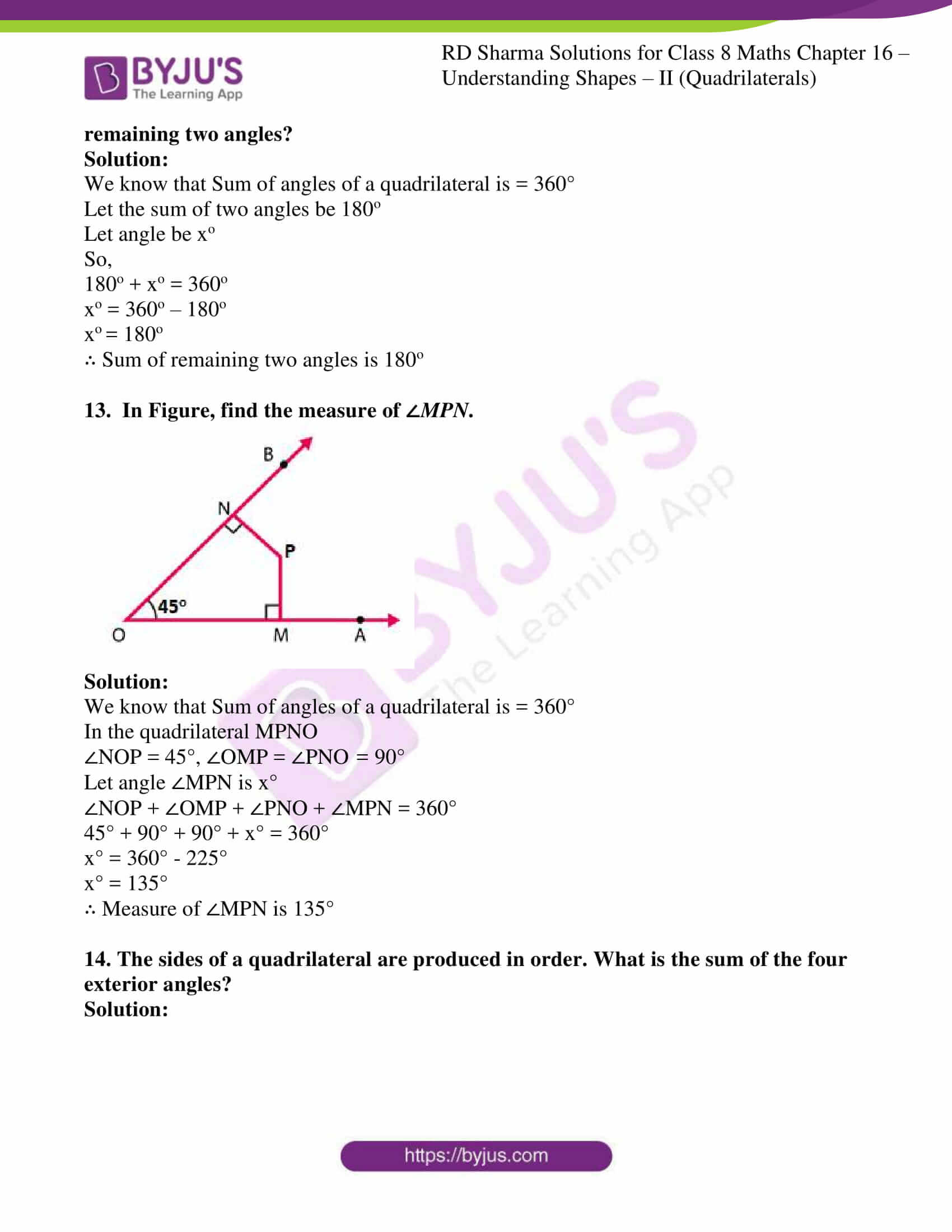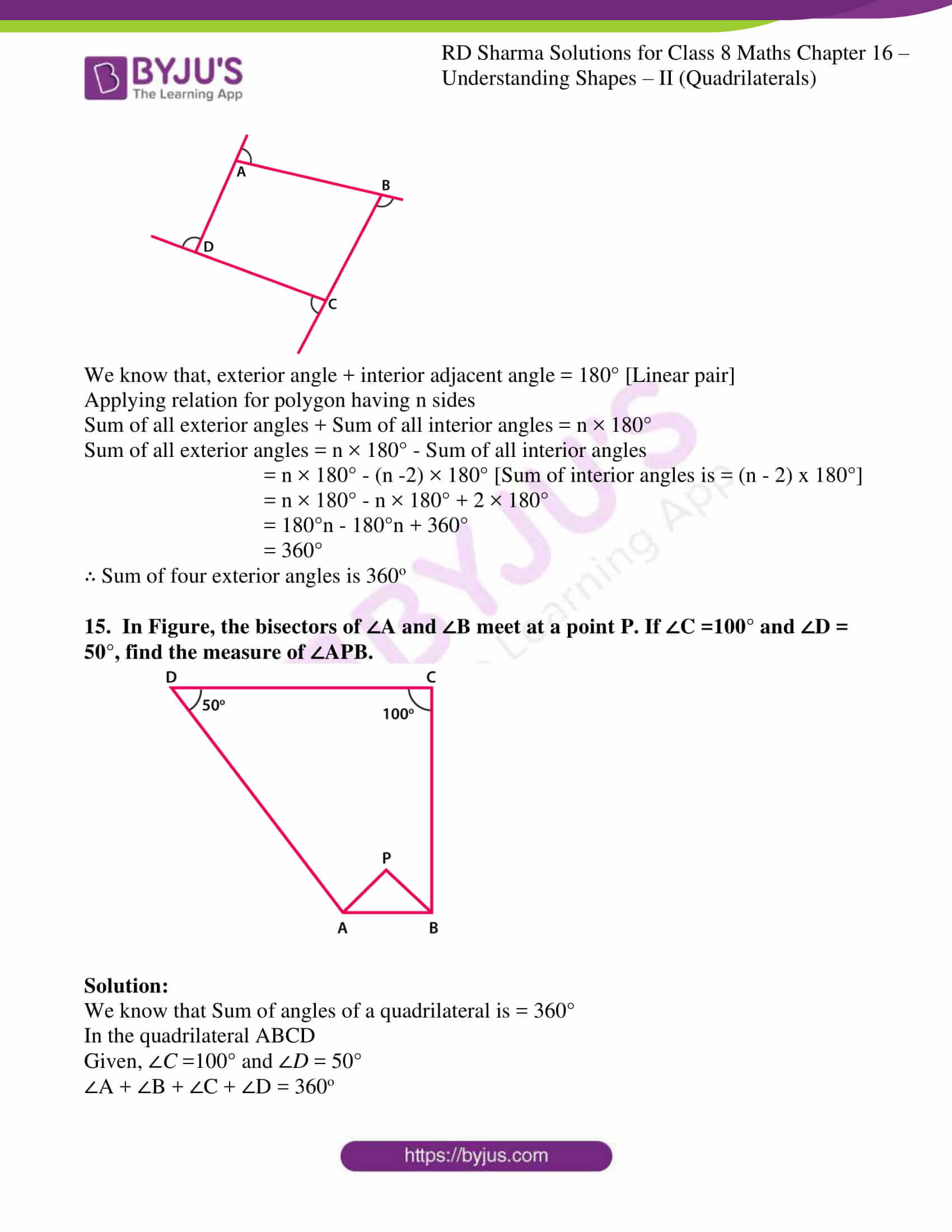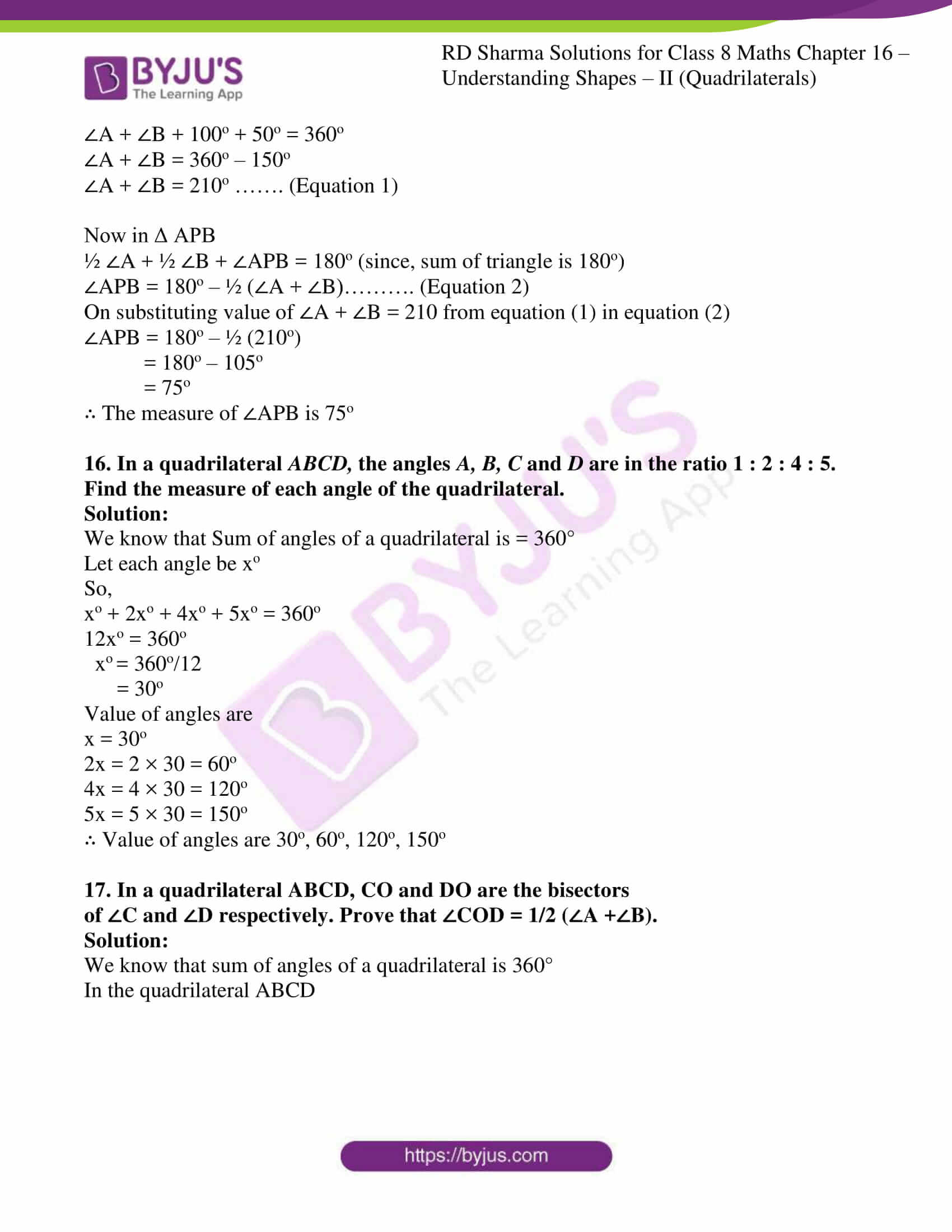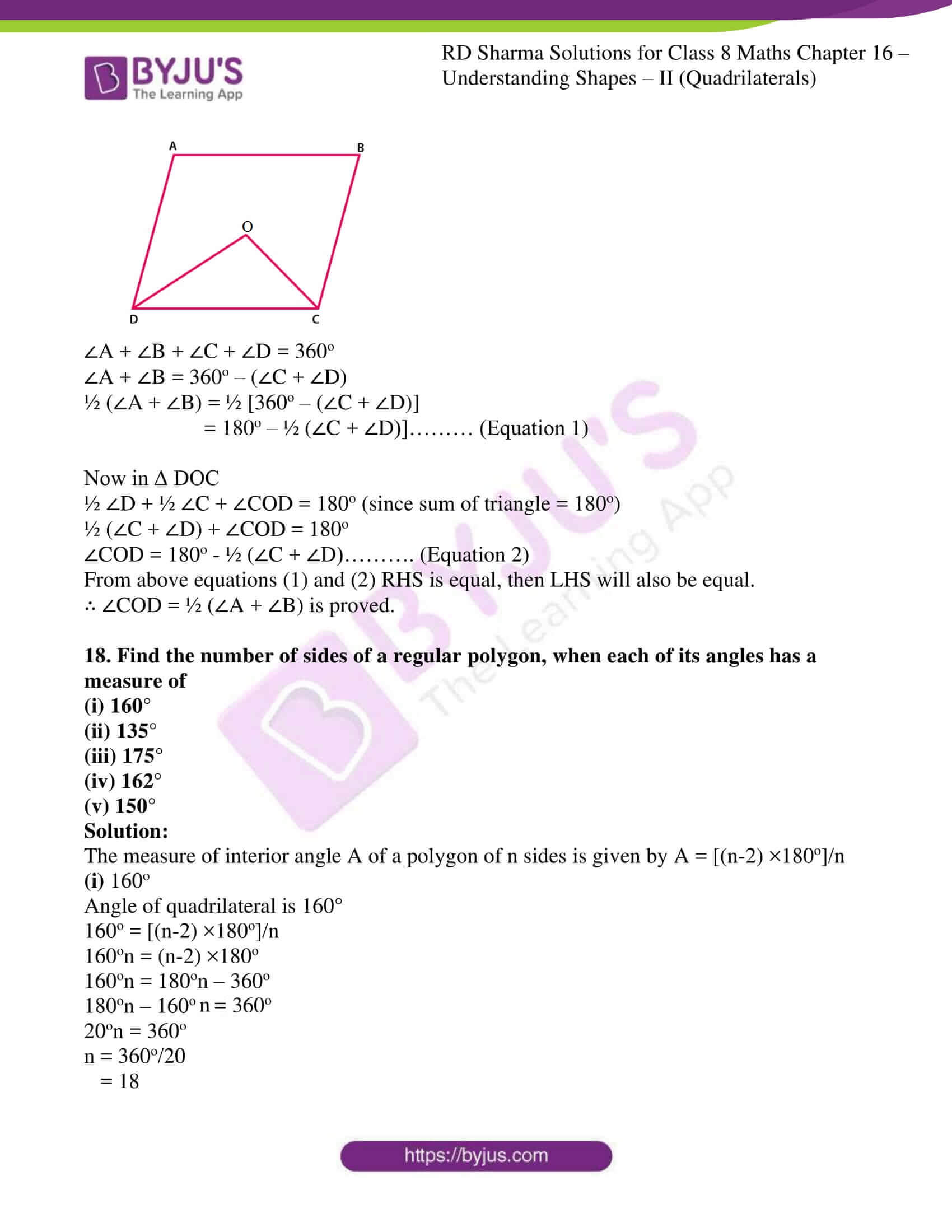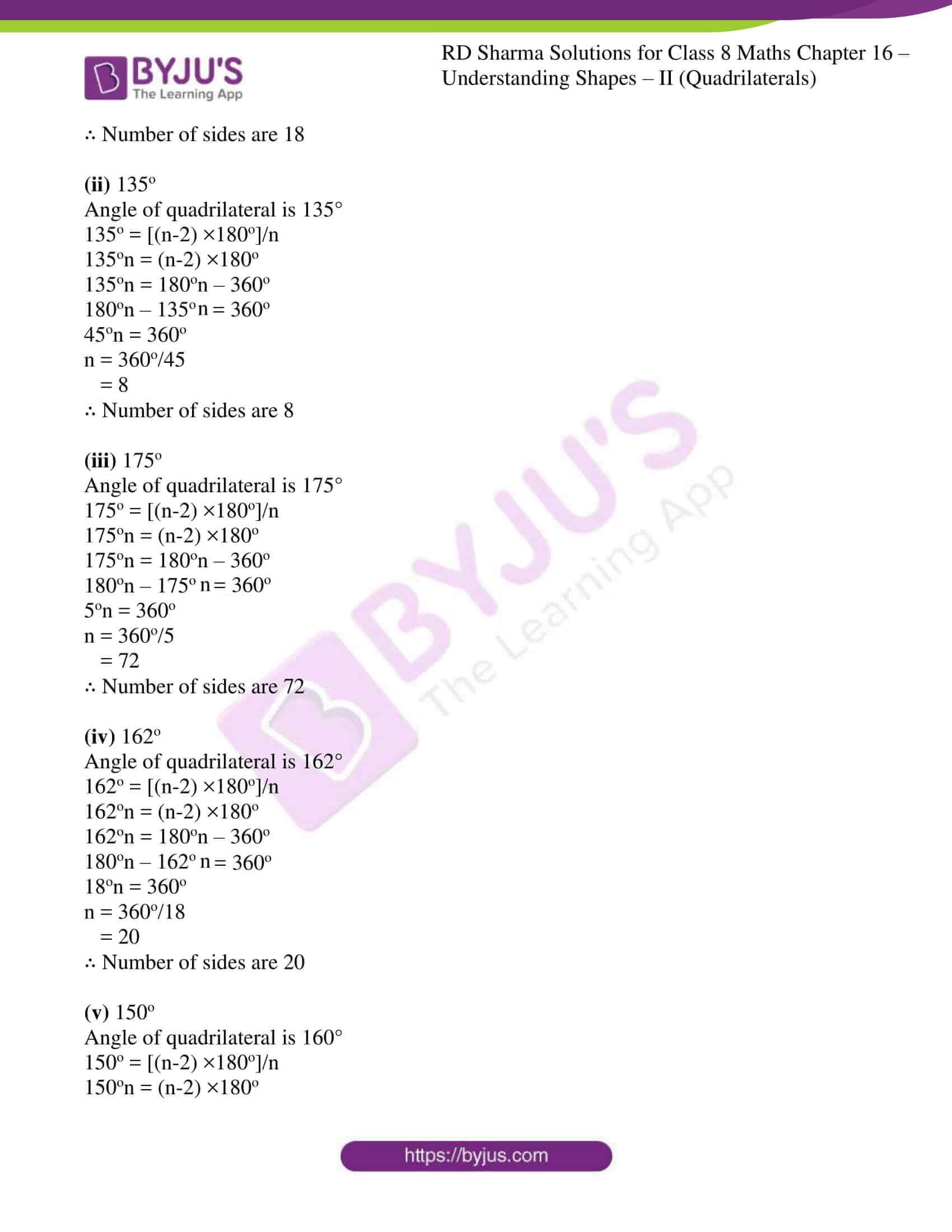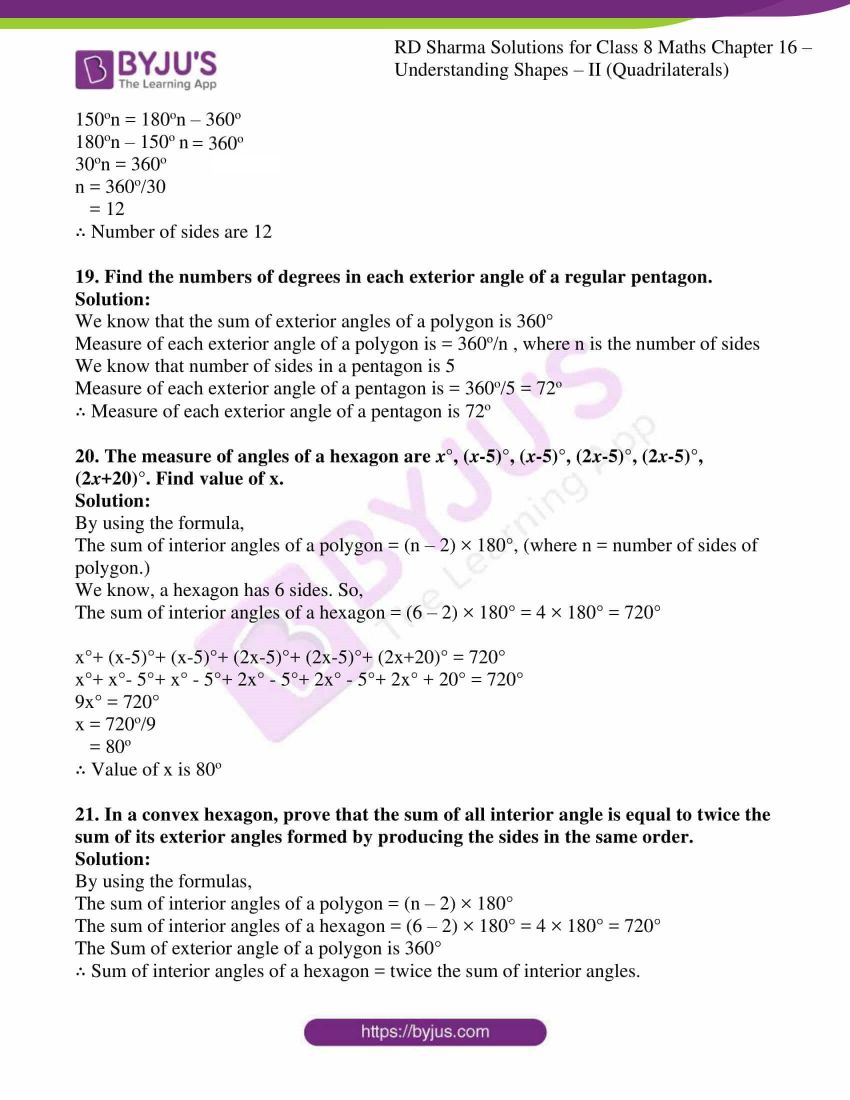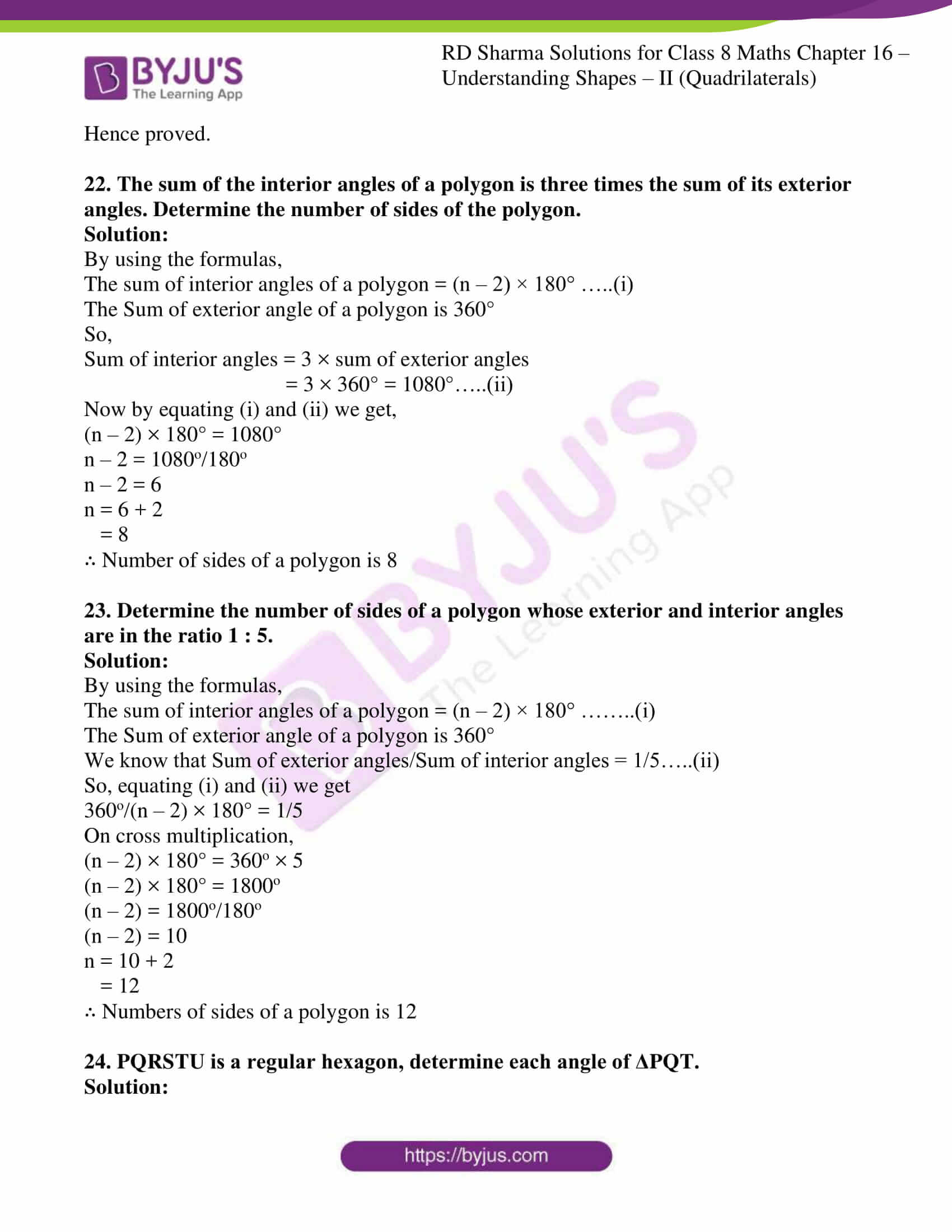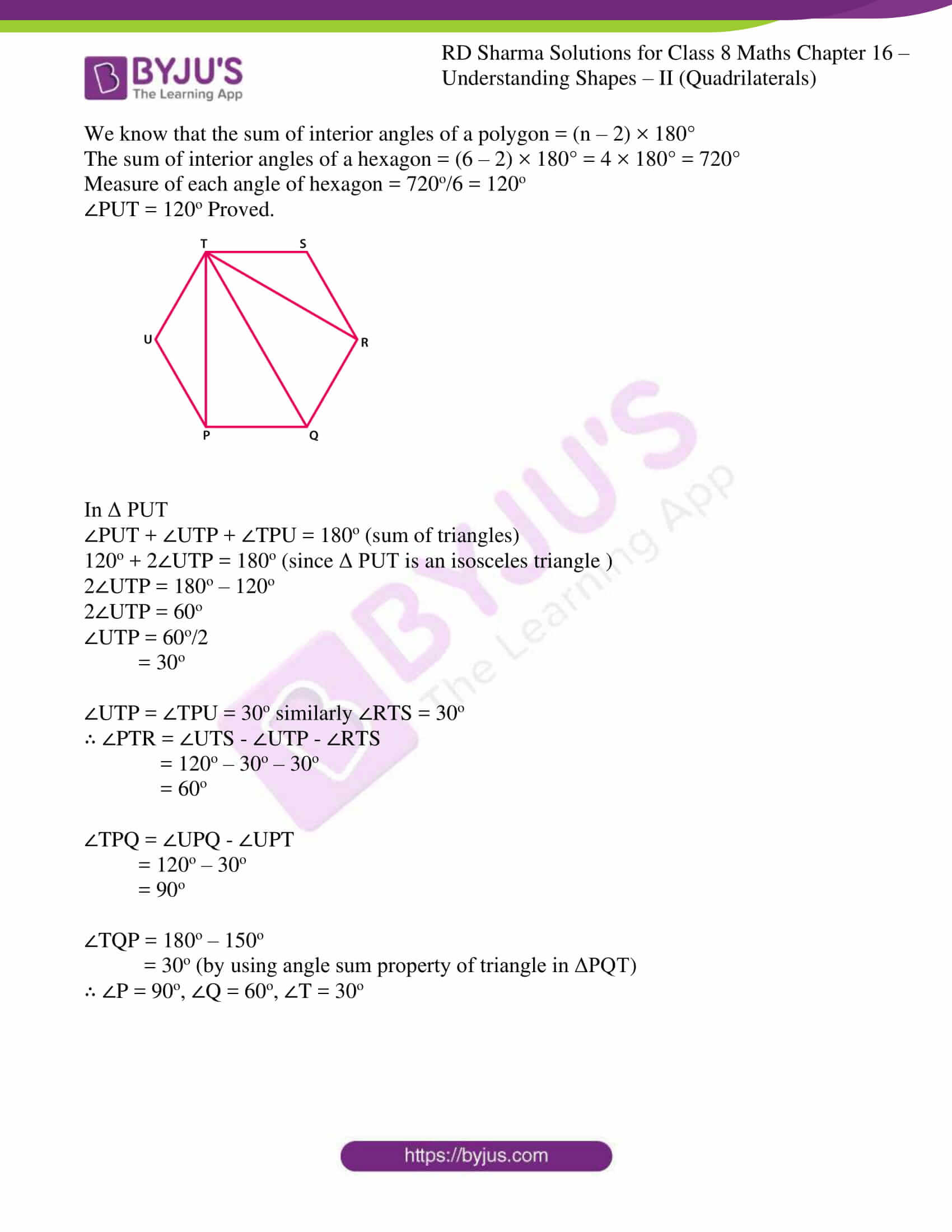### Access answers to Maths RD Sharma Solutions For Class 8 Chapter 16 Understanding Shapes- II (Quadrilaterals)

EXERCISE 16.1 PAGE NO: 16.15

1. Define the following terms:

Solution:

Definition: Let A, B, C and D be four points in a plane such that: (a) no three of them are collinear. (b) The line segments AB, BC, CD and DA do not intersect except at their end points. Then an Enclosed figure with four sides is termed as Quadrilateral.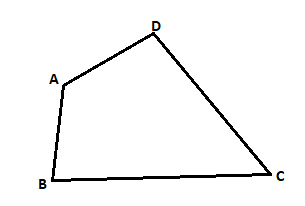Definition: If the line containing any side of the quadrilateral has the remaining vertices on the same side of it is termed as Convex Quadrilateral.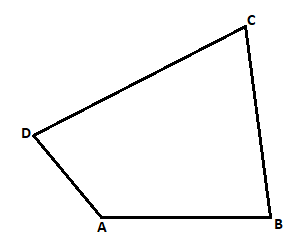Vertices A, B lie on the same side of line CD, vertices B, C lie on the same side of line DA, vertices C, D lie on the same side of line AB, vertices D, A lie on the same side of line BC.

2. In a quadrilateral, define each of the following:
(i) Sides
(ii) Vertices
(iii) Angles
(iv) Diagonals
(vii) Opposite sides
(viii) Opposite angles
(ix) Interior
(x) Exterior

Solution: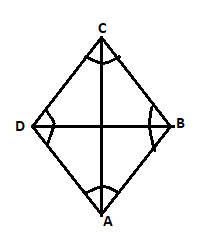(i) Sides: In a quadrilateral. All the sides may have same of different length.

The four line segments AB, BC, CD and DA are called its sides.

(ii) Vertices

Vertices are the angular points where two sides or edges meet.

A, B, C and D are the four vertices in a quadrilateral.

(iii) Angles

Angle is the inclination between two sides of a quadrilateral. i.e. meeting point of two sides is an angle. ABC, BCA, CDA and DAB are the four angles in a quadrilateral.

(iv) Diagonals

The lines joining two opposite vertices is called the diagonals in a quadrilateral.

BD and AC are the two diagonals.

Angles having one common arm onto the sides is called the adjacent angles.

When two sides have common endpoint is termed as adjacent sides.

AB BC, BC CA, CD DA, DA AB are pairs of adjacent sides in a quadrilateral.

(vii) Opposite sides: Opposite sides when they don’t meet at any point is termed as opposite sides.

AB CD, BC DA are the pairs of opposite sides in a quadrilateral.

(viii) Opposite angles

Two angles, which are not adjacent angles are termed as opposite angles.

A and C, angles B and D are opposite angles in a quadrilateral.

(ix) Interior

The part of plane when points are enclosed within the quadrilateral is called as interior.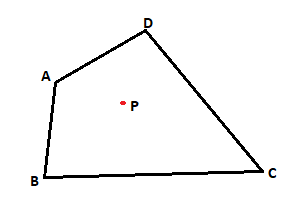(x) Exterior

The part of plane when points are not enclosed within the quadrilateral is called as exterior.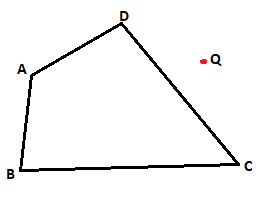3. Complete each of the following, so as to make a true statement:
(i) A quadrilateral has ________ sides.
(iii) A quadrilateral has ________, no three of which are ________.
(v) The number of pairs of adjacent angles of a quadrilateral is ________.
(vi) The number of pairs of opposite angles of a quadrilateral is ________.
(vii) The sum of the angles of a quadrilateral is ________.
(viii) A diagonal of a quadrilateral is a line segment that joins two ________ vertices of the quadrilateral.
(ix) The sum of the angles of a quadrilateral is ________ right angles.
(x) The measure of each angle of a convex quadrilateral is ________ 180°.
(xi) In a quadrilateral the point of intersection of the diagonals lies in ________ of the quadrilateral.
(xii) A point is in the interior of a convex quadrilateral, if it is in the ________ of its two opposite angles.
(xiii) A quadrilateral is convex if for each side, the remaining ________ lie on the same side of the line containing the side.

Solution:

(i) A quadrilateral has four sides.

(ii) A quadrilateral has four angles.

(iii) A quadrilateral has four, no three of which are collinear.

(iv) A quadrilateral has two diagonals.

(v) The number of pairs of adjacent angles of a quadrilateral is four.

(vi) The number of pairs of opposite angles of a quadrilateral is two.

(vii) The sum of the angles of a quadrilateral is 3600.

(viii) A diagonal of a quadrilateral is a line segment that joins two opposite vertices of the quadrilateral.

(ix) The sum of the angles of a quadrilateral is four right angles.

(x) The measure of each angle of a convex quadrilateral is less than 180°.

(xi) In a quadrilateral the point of intersection of the diagonals lies in interior of the quadrilateral.

(xii) A point is in the interior of a convex quadrilateral, if it is in the interiors of its two opposite angles.

(xiii) A quadrilateral is convex if for each side, the remaining vertices lie on the same side of the line containing the side.

4. In Fig. 16.19, ABCD is a quadrilateral.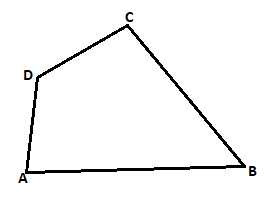(i) Name a pair of adjacent sides.
(ii) Name a pair of opposite sides.
(iii) How many pairs of adjacent sides are there?
(iv) How many pairs of opposite sides are there?
(v) Name a pair of adjacent angles.
(vi) Name a pair of opposite angles.
(vii) How many pairs of adjacent angles are there?
(viii) How many pairs of opposite angles are there?

Solution:

(i) Name a pair of adjacent sides.

Adjacent sides are: AB, BC or BC, CD or CD, DA or AD, AB

(ii) Name a pair of opposite sides.

opposite sides are: AB, CD or BC, DA

(iii) How many pairs of adjacent sides are there?

Four pairs of adjacent sides i.e. AB BC, BC CD, CD DA and DA AB

(iv) How many pairs of opposite sides are there?

Two pairs of opposite sides. AB, DC and DA, BC

(v) Name a pair of adjacent angles.

Four pairs of Adjacent angles are: D∠AB A∠BC, A∠BC B∠CA, B∠CA C∠DA or C∠DA D∠AB

(vi) Name a pair of opposite angles.

Four pair of opposite angles are: D∠AB B∠CA and A∠BC C∠DA

(vii) How many pairs of adjacent angles are there?

Four pairs of adjacent angles. D∠AB A∠BC, A∠BC B∠CA, B∠CA C∠DA and C∠DA D∠AB

(viii) How many pairs of opposite angles are there?

Two pairs of opposite angles. D∠AB B∠CA and A∠BC C∠DA

5. The angles of a quadrilateral are 110°, 72°, 55° and x°. Find the value of x.

Solution:

We know that Sum of angles of a quadrilateral is = 360°

So,

110° + 72° + 55° + x° = 360°

x° = 360° – 237°

x° = 123o

∴ Value of x is 123o

6. The three angles of a quadrilateral are respectively equal to 110°, 50° and 40°. Find its fourth angle.

Solution:

We know that Sum of angles of a quadrilateral is = 360°

So,

110° + 50° + 40° + x° = 360°

x° = 360° – 200°

x° = 160o

∴ Value of fourth angle is 160o

7. A quadrilateral has three acute angles each measures 80°. What is the measure of the fourth angle?

Solution:

We know that Sum of angles of a quadrilateral is = 360°

So,

80° + 80° + 80° + x° = 360°

x° = 360° – 240°

x° = 120o

∴ Value of fourth angle is 120o

8. A quadrilateral has all its four angles of the same measure. What is the measure of each?

Solution:

We know that Sum of angles of a quadrilateral is = 360°

Let each angle be xo

So,

xo + xo + xo + xo = 360o

xo = 360o/4

= 90o

∴ Value of angle is 90o each.

9. Two angles of a quadrilateral are of measure 65° and the other two angles are equal. What is the measure of each of these two angles?

Solution:

We know that Sum of angles of a quadrilateral is = 360°

Let each angle be xo

So,

65o + 65o + xo + xo = 360o

2xo = 360o – 130o

xo = 230o/2

= 115o

∴ Value of two angles is 115o each.

10. Three angles of a quadrilateral are equal. Fourth angle is of measure 150°. What is the measure of equal angles?

Solution:

We know that Sum of angles of a quadrilateral is = 360°

Let each angle be xo

So,

150o + xo + xo + xo = 360o

3xo = 360o – 150o

xo = 210o/3

= 70o

∴ Value of equal angles is 70o each.

11. The four angles of a quadrilateral are as 3 : 5 : 7 : 9. Find the angles.

Solution:

We know that Sum of angles of a quadrilateral is = 360°

Let each angle be xo

So,

3xo + 5xo + 7xo + 9xo = 360o

24xo = 360o

xo = 360o/24

= 15o

Value of angles are

3x = 3 × 15 = 45o

5x = 5 × 15 = 75o

7x = 7 × 15 = 105o

9x = 9 × 15 = 135o

∴ Value of angles are 45o, 75o, 105o, 135o

12. If the sum of the two angles of a quadrilateral is 180°. What is the sum of the remaining two angles?

Solution:

We know that Sum of angles of a quadrilateral is = 360°

Let the sum of two angles be 180o

Let angle be xo

So,

180o + xo = 360o

xo = 360o – 180o

xo = 180o

∴ Sum of remaining two angles is 180o

13. In Figure, find the measure of ∠MPN.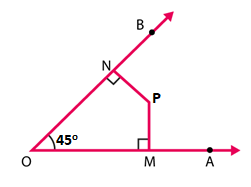Solution:

We know that Sum of angles of a quadrilateral is = 360°

∠NOP = 45°, ∠OMP = ∠PNO = 90°

Let angle ∠MPN is x°

∠NOP + ∠OMP + ∠PNO + ∠MPN = 360°

45° + 90° + 90° + x° = 360°

x° = 360° – 225°

x° = 135°

∴ Measure of ∠MPN is 135°

14. The sides of a quadrilateral are produced in order. What is the sum of the four exterior angles?

Solution: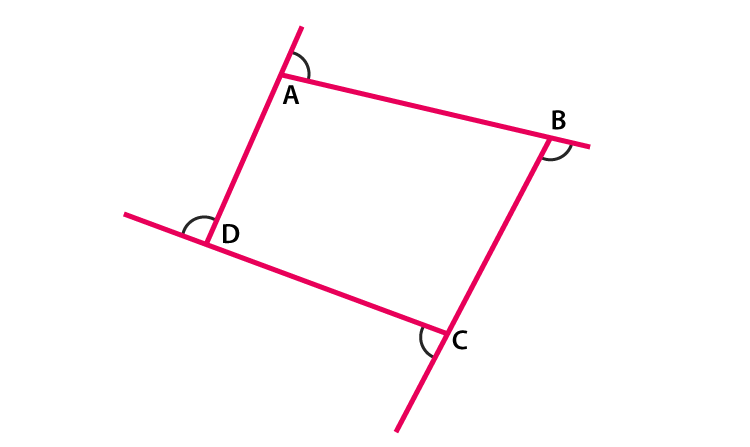We know that, exterior angle + interior adjacent angle = 180° [Linear pair]

Applying relation for polygon having n sides

Sum of all exterior angles + Sum of all interior angles = n × 180°

Sum of all exterior angles = n × 180° – Sum of all interior angles

= n × 180° – (n -2) × 180° [Sum of interior angles is = (n – 2) x 180°]

= n × 180° – n × 180° + 2 × 180°

= 180°n – 180°n + 360°

= 360°

∴ Sum of four exterior angles is 360o

15. In Figure, the bisectors of ∠A and ∠B meet at a point P. If ∠C =100° and ∠D = 50°, find the measure of ∠APB.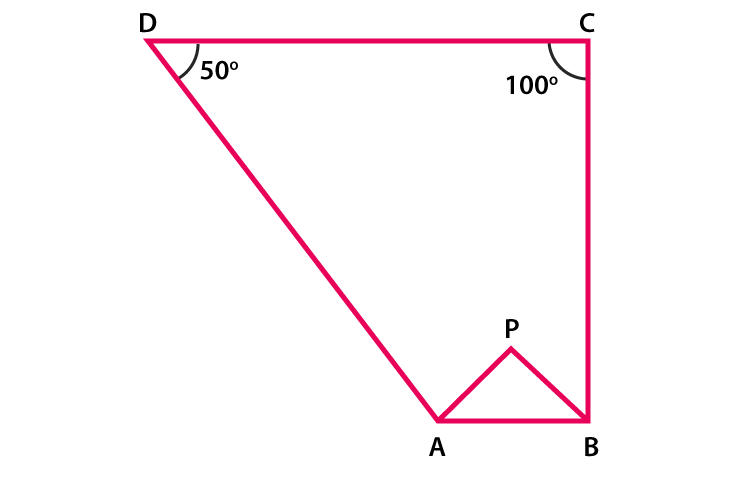Solution:

We know that Sum of angles of a quadrilateral is = 360°

Given, ∠C =100° and ∠D = 50°

∠A + ∠B + ∠C + ∠D = 360o

∠A + ∠B + 100o + 50o = 360o

∠A + ∠B = 360o – 150o

∠A + ∠B = 210o ……. (Equation 1)

Now in Δ APB

½ ∠A + ½ ∠B + ∠APB = 180o (since, sum of triangle is 180o)

∠APB = 180o – ½ (∠A + ∠B)………. (Equation 2)

On substituting value of ∠A + ∠B = 210 from equation (1) in equation (2)

∠APB = 180o – ½ (210o)

= 180o – 105o

= 75o

∴ The measure of ∠APB is 75o

16. In a quadrilateral ABCD, the angles A, B, C and D are in the ratio 1 : 2 : 4 : 5. Find the measure of each angle of the quadrilateral.

Solution:

We know that Sum of angles of a quadrilateral is = 360°

Let each angle be xo

So,

xo + 2xo + 4xo + 5xo = 360o

12xo = 360o

xo = 360o/12

= 30o

Value of angles are

x = 30o

2x = 2 × 30 = 60o

4x = 4 × 30 = 120o

5x = 5 × 30 = 150o

∴ Value of angles are 30o, 60o, 120o, 150o

17. In a quadrilateral ABCD, CO and DO are the bisectors of ∠C and ∠D respectively. Prove that ∠COD = 1/2 (∠A +∠B).

Solution:

We know that sum of angles of a quadrilateral is 360°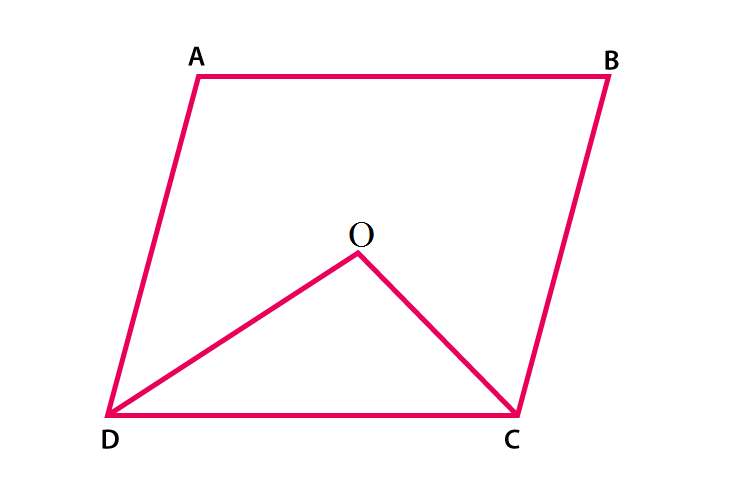∠A + ∠B + ∠C + ∠D = 360o

∠A + ∠B = 360o – (∠C + ∠D)

½ (∠A + ∠B) = ½ [360o – (∠C + ∠D)]

= 180o – ½ (∠C + ∠D)]……… (Equation 1)

Now in Δ DOC

½ ∠D + ½ ∠C + ∠COD = 180o (since sum of triangle = 180o)

½ (∠C + ∠D) + ∠COD = 180o

∠COD = 180o – ½ (∠C + ∠D)………. (Equation 2)

From above equations (1) and (2) RHS is equal, then LHS will also be equal.

∴ ∠COD = ½ (∠A + ∠B) is proved.

18. Find the number of sides of a regular polygon, when each of its angles has a measure of
(i) 160°
(ii) 135°
(iii) 175°
(iv) 162°
(v) 150°

Solution:

The measure of interior angle A of a polygon of n sides is given by A = [(n-2) ×180o]/n

(i) 160o

160o = [(n-2) ×180o]/n

160on = (n-2) ×180o

160on = 180on – 360o

180on – 160on = 360o

20on = 360o

n = 360o/20

= 18

∴ Number of sides are 18

(ii) 135o

135o = [(n-2) ×180o]/n

135on = (n-2) ×180o

135on = 180on – 360o

180on – 135on = 360o

45on = 360o

n = 360o/45

= 8

∴ Number of sides are 8

(iii) 175o

175o = [(n-2) ×180o]/n

175on = (n-2) ×180o

175on = 180on – 360o

180on – 175on = 360o

5on = 360o

n = 360o/5

= 72

∴ Number of sides are 72

(iv) 162o

162o = [(n-2) ×180o]/n

162on = (n-2) ×180o

162on = 180on – 360o

180on – 162on = 360o

18on = 360o

n = 360o/18

= 20

∴ Number of sides are 20

(v) 150o

150o = [(n-2) ×180o]/n

150on = (n-2) ×180o

150on = 180on – 360o

180on – 150on = 360o

30on = 360o

n = 360o/30

= 12

∴ Number of sides are 12

19. Find the numbers of degrees in each exterior angle of a regular pentagon.

Solution:

We know that the sum of exterior angles of a polygon is 360°

Measure of each exterior angle of a polygon is = 360o/n , where n is the number of sides

We know that number of sides in a pentagon is 5

Measure of each exterior angle of a pentagon is = 360o/5 = 72o

∴ Measure of each exterior angle of a pentagon is 72o

20. The measure of angles of a hexagon are x°, (x-5)°, (x-5)°, (2x-5)°, (2x-5)°, (2x+20)°. Find value of x.

Solution:

By using the formula,

The sum of interior angles of a polygon = (n – 2) × 180°, (where n = number of sides of polygon.)

We know, a hexagon has 6 sides. So,

The sum of interior angles of a hexagon = (6 – 2) × 180° = 4 × 180° = 720°

x°+ (x-5)°+ (x-5)°+ (2x-5)°+ (2x-5)°+ (2x+20)° = 720°

x°+ x°- 5°+ x° – 5°+ 2x° – 5°+ 2x° – 5°+ 2x° + 20° = 720°

9x° = 720°

x = 720o/9

= 80o

∴ Value of x is 80o

21. In a convex hexagon, prove that the sum of all interior angle is equal to twice the sum of its exterior angles formed by producing the sides in the same order.

Solution:

By using the formulas,

The sum of interior angles of a polygon = (n – 2) × 180°

The sum of interior angles of a hexagon = (6 – 2) × 180° = 4 × 180° = 720°

The Sum of exterior angle of a polygon is 360°

∴ Sum of interior angles of a hexagon = twice the sum of interior angles.

Hence proved.

22. The sum of the interior angles of a polygon is three times the sum of its exterior angles. Determine the number of sides of the polygon.

Solution:

By using the formulas,

The sum of interior angles of a polygon = (n – 2) × 180° …..(i)

The Sum of exterior angle of a polygon is 360°

So,

Sum of interior angles = 3 × sum of exterior angles

= 3 × 360° = 1080°…..(ii)

Now by equating (i) and (ii) we get,

(n – 2) × 180° = 1080°

n – 2 = 1080o/180o

n – 2 = 6

n = 6 + 2

= 8

∴ Number of sides of a polygon is 8

23. Determine the number of sides of a polygon whose exterior and interior angles are in the ratio 1 : 5.

Solution:

By using the formulas,

The sum of interior angles of a polygon = (n – 2) × 180° ……..(i)

The Sum of exterior angle of a polygon is 360°

We know that Sum of exterior angles/Sum of interior angles = 1/5…..(ii)

So, equating (i) and (ii) we get

360o/(n – 2) × 180° = 1/5

On cross multiplication,

(n – 2) × 180° = 360o × 5

(n – 2) × 180° = 1800o

(n – 2) = 1800o/180o

(n – 2) = 10

n = 10 + 2

= 12

∴ Numbers of sides of a polygon is 12

24. PQRSTU is a regular hexagon, determine each angle of ΔPQT.

Solution:

We know that the sum of interior angles of a polygon = (n – 2) × 180°

The sum of interior angles of a hexagon = (6 – 2) × 180° = 4 × 180° = 720°

Measure of each angle of hexagon = 720o/6 = 120o

∠PUT = 120o Proved.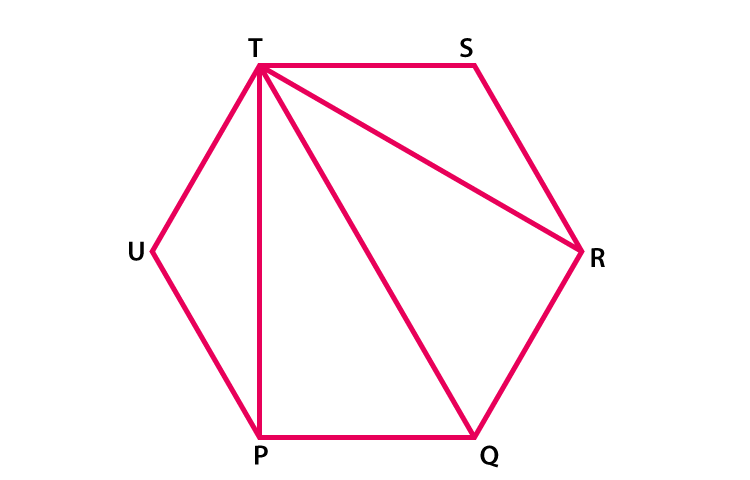In Δ PUT

∠PUT + ∠UTP + ∠TPU = 180o (sum of triangles)

120o + 2∠UTP = 180o (since Δ PUT is an isosceles triangle )

2∠UTP = 180o – 120o

2∠UTP = 60o

∠UTP = 60o/2

= 30o

∠UTP = ∠TPU = 30o similarly ∠RTS = 30o

∴ ∠PTR = ∠UTS – ∠UTP – ∠RTS

= 120o – 30o – 30o

= 60o

∠TPQ = ∠UPQ – ∠UPT

= 120o – 30o

= 90o

∠TQP = 180o – 150o

= 30o (by using angle sum property of triangle in ΔPQT)

∴ ∠P = 90o, ∠Q = 60o, ∠T = 30o